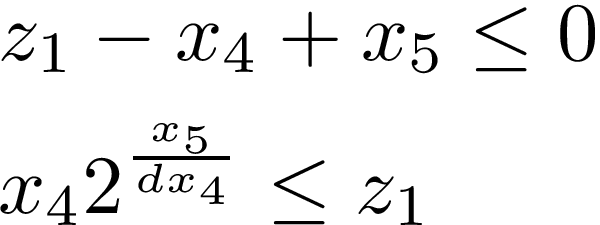# How to write these constraints with DCP rule to use in CVX

I have the following constraints in an optimization problem if you can please help me on how to write them with disciplined convex programming rule to use in CVX

Here, x_1, x_2, x_3, x_4, x_5, a, b, c, d >0.

Based on some readings in this forum and suggestion by Mark. Stone, I can divide the constraint B into two constraints as follows:Here, the last constraint I can write as follows:

Is this the correct way to handle the constraint B?

Also, how should I handle constraint A, I can do the following:

But, I am not sure what to do next.

`x(4)*2^(x(5)/d/x(4)) <= z`
`x(4)*exp(x(5)/d/x(4)*log(2)) <= z`

So constraint B can be handled with

``````variable z
z  - x(4) + x(5) <= 0
{x(5)*log(2)/d,x(4),z} == exponential(1)
``````

The exponnetial91) constraint implements the exponential cone constraint. It is listed in section 9.4 of the CVX Users’ Guide http://cvxr.com/cvx/doc/funcref.html#sets as `exp_cone` , but it is actually exponential(1) .

I will defer to a more clever person for constraint A, which I believe is convex when `a,b,c,x(1),x(2) >= 0.`

1 Like

Many thanks.
yes, a, b, c, x(1), x(2) >=0.

I do not find how to solve for constraint B, need some assistance.

By change of variables constraint A is equivalent to

t\geq x \log(1+e^{y/x}).

This we recognize as the perspective function of the softplus function \log(1+e^x) . We know that the perspective can be modeled with the same types of cones as the original function . Putting the formulation of softplus from  together with the recipe of  (actually, this is exactly example 7.1 in ) we reformulate the above constraint as

x\geq x\exp(-t/x) + x\exp((y-t)/x)

or in other words

x=x_1+x_2
x_1\geq x\exp(-t/x)
x_2\geq x\exp((y-t)/x)

with two exponential cones.

2 Likes

@Michal_Adamaszek Yeah, I was derelict in my duty for not looking carefully art the Mosek Modeling Cookbook to see the solution for that one.